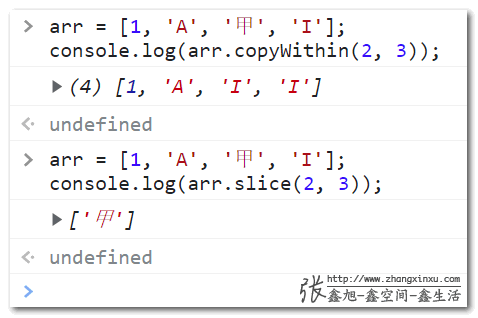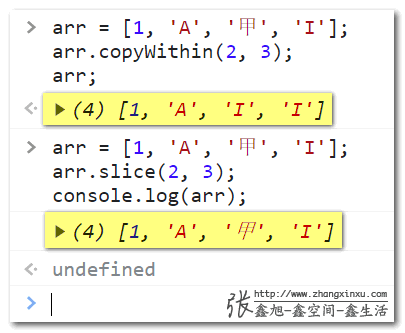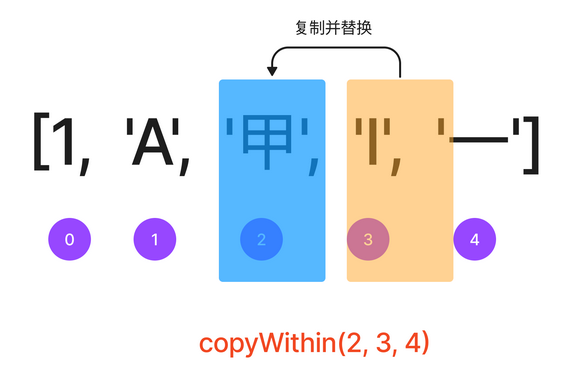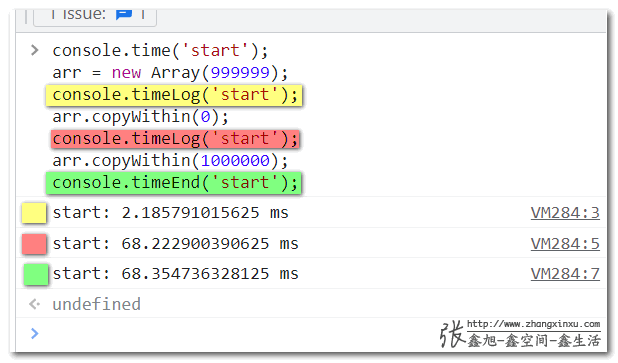## JS数组的copyWithin()语法我看了好几遍才懂### 一、copyWithin()对比slice()

JavaScript中数组的copyWithin()和slice()方法作用都是复制数组，且都是浅复制。

`copyWithin()`是将数组中的一部分复制并替换另外一部分，总长度是不变的，而`slice()`方法只复制，不替换，返回的数组长度是由复制的数组项目个数决定的。

```arr = [1, 'A', '甲', 'I'];
console.log(arr.copyWithin(2, 3));
// 结果是： [1, 'A', 'I', 'I']```
```arr = [1, 'A', '甲', 'I'];
console.log(arr.slice(2, 3));
// 结果是： ['甲']````copyWithin()`方法会改变原始的数组，而`slice()`方法并不会。

```arr = [1, 'A', '甲', 'I'];
arr.copyWithin(2, 3);
console.log(arr);
// 结果是： [1, 'A', 'I', 'I']```
```arr = [1, 'A', '甲', 'I'];
arr.slice(2, 3);
console.log(arr);
// 结果是：[1, 'A', '甲', 'I']````slice()`复制对于字符串也是有效的，但是`copyWithin()`方法却不支持。

```// 返回值是'angx'
('zhangxinxu').slice(2, 6)```

```// 会无情报错
('zhangxinxu').copyWithin(2, 6)```

### 二、copyWithin()的语法

`copyWithin()`的语法形式上不算复杂，但是理解起来有些费脑细胞，我也是看了好几遍才弄明白。

#### 语法

`Array.prototype.copyWithin(index, start, end)`

```[].copyWithin()
[].copyWithin(index)
[].copyWithin(index, start)
[].copyWithin(index, start, end)```

#### 参数

index

start

end

```arr = [1, 'A', '甲', 'I', '一'];
arr.copyWithin(2, 3, 4);```

index=2，则表示复制的值从arr这里开始替换。#### 参数细节

`copyWithin()`方法的参数细节比较多，毕竟数值有大有小，有正有负，情况不同，规则也会不同。

1. index参数

• `index`参数不设置也是合法的，不过你不设置该参数数组就会原路复制返回，类似于设置为 0，这样处理只会浪费性能，没有任何实际意义。

因此，`index`参数可以认为是必须参数。

• 如果index是小数，则会按照整数处理，并且是向下取整。例如：
```// 返回值是 true
[1, 2, 3].copyWithin(1.5).join() == [1, 2, 3].copyWithin(1).join()
// copyWithin(1.5)执行结果是：[1, 1, 2]```
• 如果index是负数。
• 如果index负数的绝对值很大，甚至比数组的长度还要大，则此时 index 当作 0 处理。例如：
```// 返回值是 ['zhang', 'xin', 'xu']
// 等同于 copyWithin(0)
['zhang', 'xin', 'xu'].copyWithin(-999)```
• 否则，最终的 index 值等于 index + arr.length，比方说 `[1, 2, 3].copyWithin(-1)` 就等同于 `[1, 2, 3].copyWithin(2)`
```// 结果是[1, 2, 1]
[1, 2, 3].copyWithin(-1);
// 结果同样是[1, 2, 1]
[1, 2, 3].copyWithin(2);```• 如果index的值超出了数组的长度范围，则不会有任何内容被复制。这里需要注意下，没有任何内容复制和所有内容都复制是有区别的，虽然看起来返回的都像是原数组，但其实并不是，性能这块是有着明显的差异的。

我们可以用非常简单的方法测试下，例如我们构造一个99万个项目的数组进行复制处理：

```console.time('start');
arr = new Array(999999);
console.timeLog('start');
arr.copyWithin(0);
console.timeLog('start');
arr.copyWithin(1000000);
console.timeEnd('start');```

我们在控制台跑一下上面的代码，通过观察执行时间就会发现执行copyWithin(0)用了近70ms，而执行copyWithin(1000000)的用时几乎就是0.• 如果 index 的位置在 start 之后，则复制的内容粘贴到数组长度的尾部就停止了，也就是不会增加数组的长度的：

例如：

```// 结果是 [1, 'A', '甲', 'A', '甲']
[1, 'A', '甲', 'I', '一'].copyWithin(3, 1);```

2. start参数

• start参数是可选的，如果不设置，则当作0处理，表示数组从头复制到尾部（index小于数值长度的前提下）；
• start如果是小数，会向下取整处理。例如`[].copyWithin(0, 1.9999)`等同于 `[].copyWithin(0, 1)`
• start如果是负数，则从数组的后面开始取值，等同于 start + arr.length；
• start如果是负数，同时绝对值小于 -1 * arr.length，则当作 0 处理。
• start如果超出数组的长度范围，则不会发生复制。

2. end参数

• end参数是可选的，如果不设置，则当作`array.length`处理，表示数组复制到尾部；
• end如果是小数，会向下取整处理。例如`[].copyWithin(0, 1, 2.999)`等同于 `[].copyWithin(0, 1, 2)`，例如：
```// 结果是：[2, 2, 3, 4]
[1, 2, 3, 4].copyWithin(0, 1, 2.9999)```
• end如果是负数，则从数组的后面开始取值，等同于 end + arr.length；
• end如果是负数，同时绝对值小于 -1 * arr.length，则当作 0 处理。
• end如果超出数组的长度范围，则当作`array.length`处理。
• 如果end对应的位置比start小，则不会发生复制。

### 三、copyWithin实际应用

#### 1. 删除数组中间某一项

`[].splice(index, 1);`

`[].copyWithin(index, index + 1).pop();`

```arr = ['甲', '乙', '丙', '丁'];
arr.copyWithin(1, 2).pop();
// 结果是['甲', '丙', '丁']
console.log(arr);```

#### 2. 置顶数组某一项

```// 自定义top置顶方法
Array.prototype.top = function (index) {
const value = this[index];
this.copyWithin(1, 0, index);
this = value;
};```

```arr = ['甲', '乙', '丙', '丁'];
arr.top(1);
// 结果是["乙", "甲", "丙", "丁"]
console.log(arr);```

#### 3. 模拟插入排序算法

```const insertionSort = (data, compare) => {
const arr = [...data];
let unsort_index = 1;

while (unsort_index < arr.length) {

while (arr[unsort_index] >= arr[unsort_index - 1]) unsort_index += 1;
const pick = arr[unsort_index];

let iter = 0;
while (iter < unsort_index && arr[iter] < pick) iter += 1;
arr.copyWithin(iter + 1, iter, unsort_index);
arr[iter] = pick;

unsort_index += 1;
}
return arr;
}

const input = [2, 3, 5, 1, 9, 8, 6, 6];
const asc = (a, b) => a - b;
const dsc = (a, b) => b - a;

console.log({ input, asc_sorted: insertionSort(input, asc) });
console.log({ input, dsc_sorted: insertionSort(input, dsc) });```

### 四、结束语

`copyWithin()`是一个非常高性能的数组移动方法。

😊

（本篇完）

﻿
1. MO说道：

会用了，不过暂时没有使用场景~~

2. scienceFunq说道：

用slice和splice方法实现了一个和Array.prototype.copyWithin相同语义的函数,函数复制操作部分逻辑不复杂,对参数的处理上稍微复杂一点:
function copyWithin(arr, target, start, end = arr.length){
target=Number(target);
start=Number(start);
end=Number(end);
target = Number.isNaN(target) ? 0 : Math.trunc(target);
start = Number.isNaN(start) ? 0 : Math.trunc(start);
end = Number.isNaN(end) ? 0 : Math.trunc(end);

let length = arr.length;
target = target < 0 ? length + target : target;
target = target < 0 ? 0 : target ;
start = start < 0 ? length + start : start;
start = start < 0 ? 0 : start;
end = end length ? length : end = length || start >= length || end <= start)
return arr;

let copyLength = Math.min(end – start, length – target);
let temp = arr.slice(start, copyLength + start);
arr.splice(target, temp.length, …temp);
return arr;
}